# High School Math : Angles

## Example Questions

2 Next →

### Example Question #25 : Graphs And Inverses Of Trigonometric Functions

Find a coterminal angle for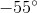Possible Answers: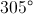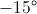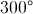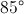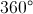Correct answer:Explanation:

Coterminal angles are angles that, when drawn in the standard position, share a terminal side. You can find these angles by adding or subtracting 360 to the given angle. Thus, the only angle measurement that works from the answers given is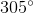### Example Question #26 : Graphs And Inverses Of Trigonometric Functions

Which of the following angles is coterminal with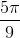?

Possible Answers: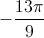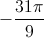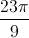Each angle given in the other choices is coterminal with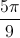.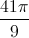Correct answer:

Each angle given in the other choices is coterminal with.

Explanation:

For an angle to be coterminal with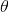, that angle must be of the form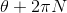for some integer- or, equivalently, the difference of the angle measures multiplied by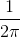must be an integer. We apply this test to all four choices.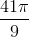: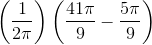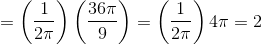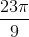: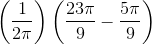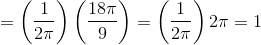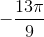: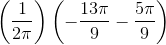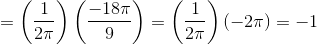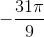: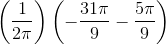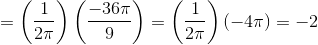All four choices pass the test, so all four angles are coterminal with.

### Example Question #21 : Trigonometry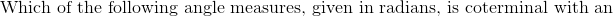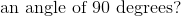Possible Answers: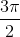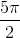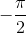Correct answer:Explanation: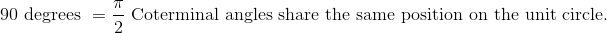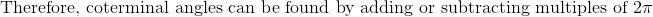.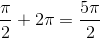### Example Question #22 : Trigonometry

Which of the following choices represents a pair of coterminal angles?

Possible Answers: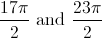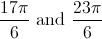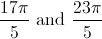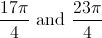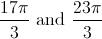Correct answer:Explanation:

For two angles to be coterminal, they must differ by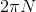for some integer- or, equivalently, the difference of the angle measures multiplied bymust be an integer. We apply this test to all five choices.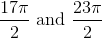: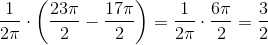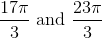: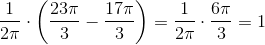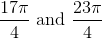: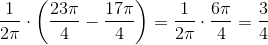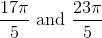: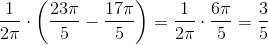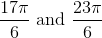: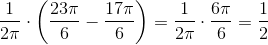The only angles that pass the test - and are therefore coterminal - are.

### Example Question #24 : Graphs And Inverses Of Trigonometric Functions

Which one of these is positive in quadrant III?

Possible Answers:

Sine

No trig functions

Tangent

All trig functions

Cosine

Correct answer:

Tangent

Explanation:

The pattern for positive functions is All Student Take Calculus. In quandrant I, all trigonometric functions are positive. In quadrant II, sine is positive. In qudrant III, tangent is positive. In quadrant IV, cosine is positive.

2 Next →

### All High School Math Resources